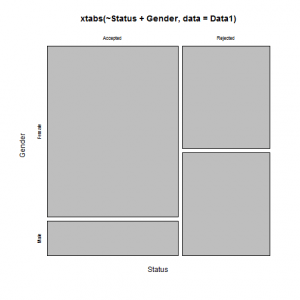Want to share your content on R-bloggers? click here if you have a blog, or here if you don't.The `xtabs()` function creates contingency tables in frequency-weighted format. Use `xtabs()` when you want to numerically study the distribution of one categorical variable, or the relationship between two categorical variables. Categorical variables are also called “factor” variables in R.

Using a formula interface, `xtabs()` can create a contingency table, (also a “sparse matrix”), from cross-classifying factors, usually contained in a data frame.

Answers to the exercises are available here.

Exercise 1
`xtabs()` with One Categorical Variable

Input the following required Data Frame:
``` Data1 <- data.frame(Reference = c("KRXH", "KRPT", "FHRA", "CZKK", "CQTN", "PZXW", "SZRZ", "RMZE", "STNX", "TMDW"), Status = c("Accepted", "Accepted", "Rejected", "Accepted", "Rejected", "Accepted", "Rejected", "Rejected", "Accepted", "Accepted"), Gender = c("Female", "Male", "Male", "Female", "Female", "Female", "Male", "Female", "Female", "Female"), Test = c("Test1", "Test1", "Test2", "Test3", "Test1", "Test4", "Test4", "Test2", "Test3", "Test1"), NewOrFollowUp = c("New", "New", "New", "New", "New", "Follow-up", "New", "New", "New", "New")) ```

The `xtabs()` function can display the frequency, or count, of the levels of categorical variables. For the first exercise, use the `xtabs()` function to find the count of levels in the variable, “`Status`“, within the above dataframe, “`Data1`“.

Exercise 2
Two Categorical Variables – Discoving relationships within a dataset

Next, using the `xtabs()` function, apply two variables from “`Data1`“, to create a table delineating the relationship between the “`Reference`” category, and the “`Status`” category.

Exercise 3
Three Categorical Variables – Creating a Multi-Dimensional Table

Apply three variables from “`Data1`” to create a Multi-Dimensional Cross-Tabulation of “`Status`“, “`Gender`“, and “`Test`“.

Exercise 4
Creating Two Dimensional Tables from Multi-Dimensional
Cross-Tabulations

Enclose the `xtabs()` formula from Exercise 3 within the “`ftable()`” function, to display a Multi-Dimensional Cross-Tabulation in two dimensions.

Exercise 5
Row Percentages

The R package “`tigerstats`” is required for the next two exercises.

```if(!require(tigerstats)) {install.packages("tigerstats"); require(tigerstats)} library(tigerstats)```

1) Create an `xtabs()` formula that cross-tabulates “`Status`“, and “`Test`“.
2) Enclose the `xtabs()` formula in the tigerstats function, “`rowPerc()`” to display row percentages for “`Status`” by “`Test`“.

Exercise 6
Column Percentages

1) Create an `xtab()` formula that cross-tabulates “`Reference`“, and “`Status`“.
2) Use “`colPerc()`” to display column percentages for “`Reference`” by “`Status`“.

Exercise 7
Plotting Cross-Tabulations

Use the “`plot()`” function, and the “`xtabs()`” function to plot “`Status`” by “`Gender`“.

Exercise 8
`xtabs()` – Explanatory and Response Variables

In order to examine whether the explanatory variable “`Gender`” affects the response variable “` Status`“, create a two factor `xtabs()` formula with the Response variable as the first condition, and the Explanatory variable as the second condition.

Exercise 9
Using `cbind()` with `xtabs()`

Using the “`cbind()`” function within an `xtabs()` formula can define the last two columns of a flat table of your dataset. The variable after ~ (tilde) will display as the row data. For example, `ftable(xtabs(cbind(variable1, variable2) ~ variable3, data=" "))`.

For this exercise, create a flat table with columns for “`Gender`” and “`Test`“. The row variables are “`Reference`“.

Exercise 10
Testing Correlation with `xtabs()`

When processed through the “`summary()`” function, an `xtabs()` formula can test for independence of variables. Therefore, use `summary()` and `xtabs()` to test for a “`Reference`” affecting “`Status`” correlation.